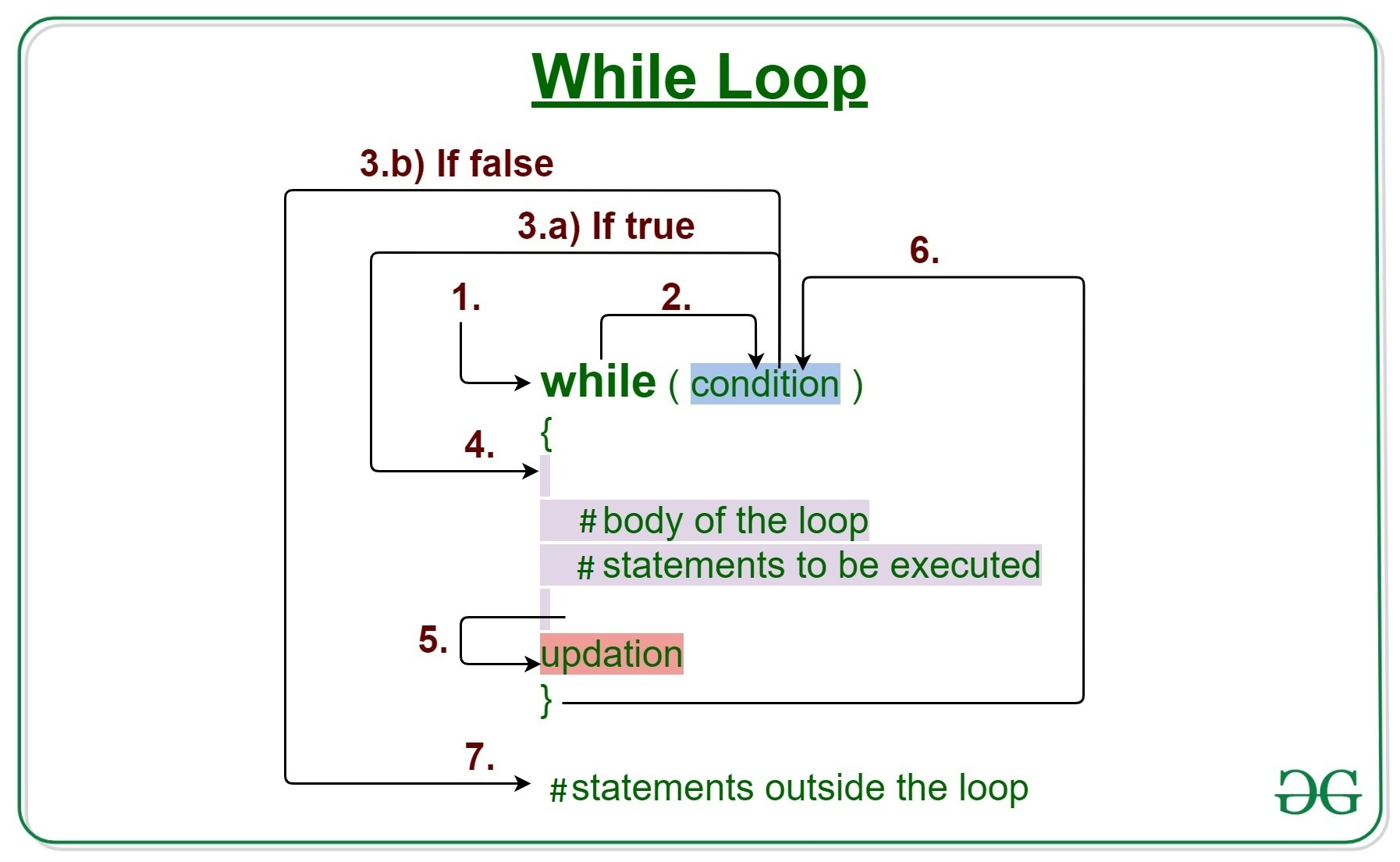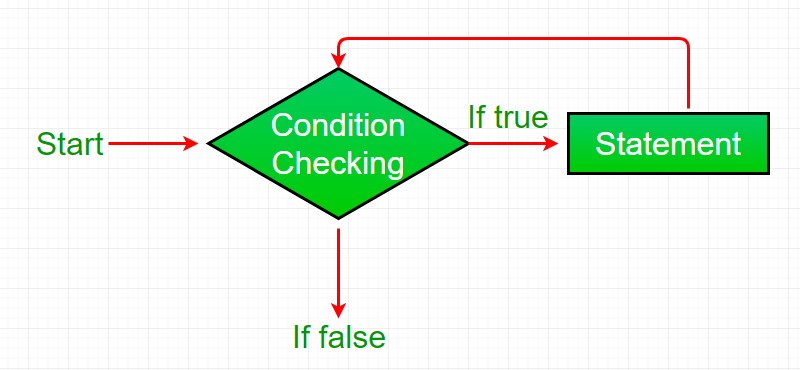Open in App
Not now

# R – while loop

• Difficulty Level : Expert
• Last Updated : 27 Oct, 2021

While loop in R programming language is used when the exact number of iterations of loop is not known beforehand. It executes the same code again and again until a stop condition is met. While loop checks for the condition to be true or false n+1 times rather than n times. This is because the while loop checks for the condition before entering the body of the loop.

### R- While loop Syntax:

```while (test_expression) {
statement
update_expression
}  ```## How does a While loop execute?

• Control falls into the while loop.
• The flow jumps to Condition
• Condition is tested.
• If Condition yields true, the flow goes into the Body.
• If Condition yields false, the flow goes outside the loop
• The statements inside the body of the loop get executed.
• Updation takes place.
• Control flows back to Step 2.
• The while loop has ended and the flow has gone outside.

## Important Points about while loop in R language:

• It seems to be that while loop will run forever but it is not true, condition is provided to stop it.
• When the condition is tested and the result is false then loop is terminated.
• And when the tested result is True, then loop will continue its execution.

## R – while loop Flowchart:Example 1:

## R

 `# R program to illustrate while loop`   `result <- ``c``(``"Hello World"``)` `i <- 1`   `# test expression ` `while ``(i < 6) {`   `   ``print``(result)` `   `  `   ``# update expression ` `   ``i = i + 1` `}`

Output:

``` "Hello World"
 "Hello World"
 "Hello World"
 "Hello World"
 "Hello World"```

Example 2:

## R

 `# R program to illustrate while loop`   `result <- 1` `i <- 1`   `# test expression ` `while ``(i < 6) {`   `   ``print``(result)` `   `  `   ``# update expression ` `   ``i = i + 1` `   ``result = result + 1` `}`

Output:

``` 1
 2
 3
 4
 5```

## R – while loop break

Here we will use the break statement in the R programming language. Break statement in R is used to bring the control out of the loop when some external condition is triggered.

## R

 `# R program to illustrate while loop`   `result <- ``c``(``"Hello World"``)` `i <- 1`   `# test expression` `while ``(i < 6) {`   `    ``print``(result)` `    `  `    ``if``( i == 3){` `        ``break``}` `    ``# update expression` `    ``i = i + 1` `}`

Output:

``` "Hello World"
 "Hello World"
 "Hello World"```

My Personal Notes arrow_drop_up
Related Articles